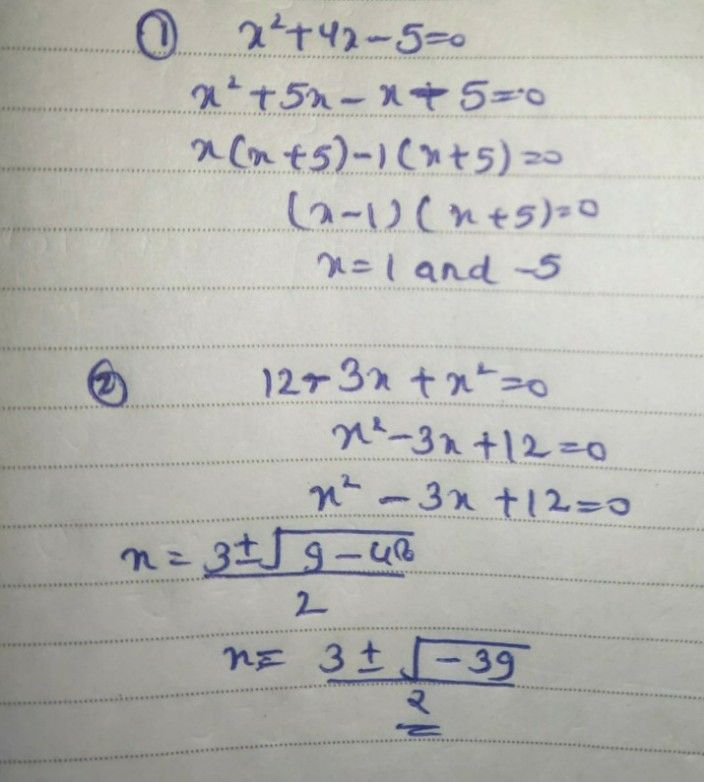Symbol
Problem$Directions:$ Transform the following mathematical sentences into quadratic inequalities by changing or adding only one element from the given. $1.$ $x^{2}+4x-5=0$ $6.$ $15-2x=x^{2}$ 2. $12-3x+x^{2}=0$ $7.$ $\left(b^{2}-1\right)\left(2b+5\right)\leq 0$ $3.$ $4.$ $4t^{3}-7t+1\geq 0$ $x^{2}+10x<x^{2}-3$ $8.$ $g^{2}\left(g+7\right)>8$ $5.$ $\left(a+4\right)\left(a+3\right)=5$ $9.$ $2h+5\geq h$ $10.3m+20>0$
7th-9th grade
Other
Search count: 107
SolutionQanda teacher - Barkha❣️